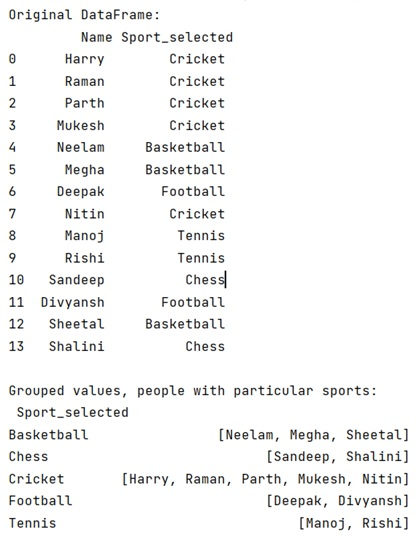# How to group DataFrame rows into list in pandas groupby?

Given a DataFrame, we have to group rows into a list.
Submitted by Pranit Sharma, on April 30, 2022

DataFrame rows are based on the index values. We can manipulate both rows and columns in pandas. On the other hand, indexes are the integer values representing the number of rows and columns separately. We can perform many operations on rows of a DataFrame based on a specific condition.

## Problem statement

Given a DataFrame, we have to group rows into a list.

## Groupping DataFrame rows into list in pandas

For this purpose, we will use the groupby() method of Pandas. This method is used to group the data inside DataFrame based on the condition passed inside it as a parameter. It works on a split and group basis. It splits the data and then combines them in the form of a series or any other sequence.

### Syntax

```DataFrame.groupby(
by=None,
axis=0,
level=None,
as_index=True,
sort=True,
group_keys=True,
squeeze=NoDefault.no_default,
observed=False,
dropna=True
)

# or
DataFrame.groupby()
```

### Parameter(s)

• by: this parameter is none by default, but it takes a map, function, string, or any other iterable object.
• axis: it is the integer value that is 0 by default.
• It has some other optional parameters like level, sort, as_index, group_keys, and squeeze.

### Return value

The method returns a groupby object.

Note

To work with pandas, we need to import pandas package first, below is the syntax:

```import pandas as pd
```

Let us understand with the help of an example.

## Python program to group DataFrame rows into list in pandas

```# Importing pandas package
import pandas as pd

# Creating a dictionary
dict = {
'Name':['Harry','Raman','Parth','Mukesh','Neelam','Megha','Deepak','Nitin','Manoj','Rishi','Sandeep','Divyansh','Sheetal','Shalini'],
}

# Creating a DataFrame
df = pd.DataFrame(dict)

# Display original DataFrame
print("Original DataFrame:\n",df,"\n")

# Grouping the column named Sport selected with the column Name
result = df.groupby('Sport_selected')['Name'].apply(list)

# Display Result
print("Grouped values, people with particular sports:\n",result)
```

### Output

The output of the above program is: